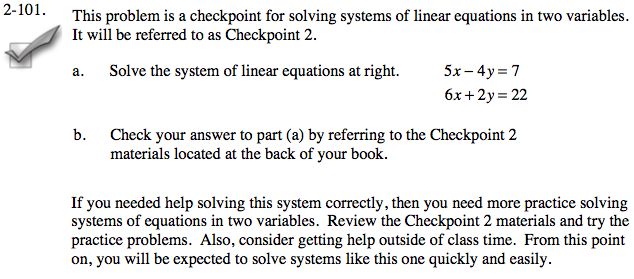### Home > AC > Chapter 14 > Lesson 14.2.1.7 > Problem2-101

2-101.
1. This problem is a checkpoint for solving systems of linear equations in two variables. It will be referred to as Checkpoint 2. Homework Help ✎1. Solve the system of linear equations below.

5x − 4y = 7
6x + 2y = 22

2. Check your answer to part (a) by referring to the Checkpoint 2 materials located at the back of your book.

If you needed help solving this system correctly, then you need more practice solving systems of equations in two variables. Review the Checkpoint 2 materials and try the practice problems. Also, consider getting help outside of class time. From this point on, you will be expected to solve systems like this one quickly and easily.Answers and extra practice are located in the back of your printed textbook or in the Reference Tab of your eBook.
If you have an eBook for A2C, login and then click the following link: Checkpoint 2 Answers and Practice